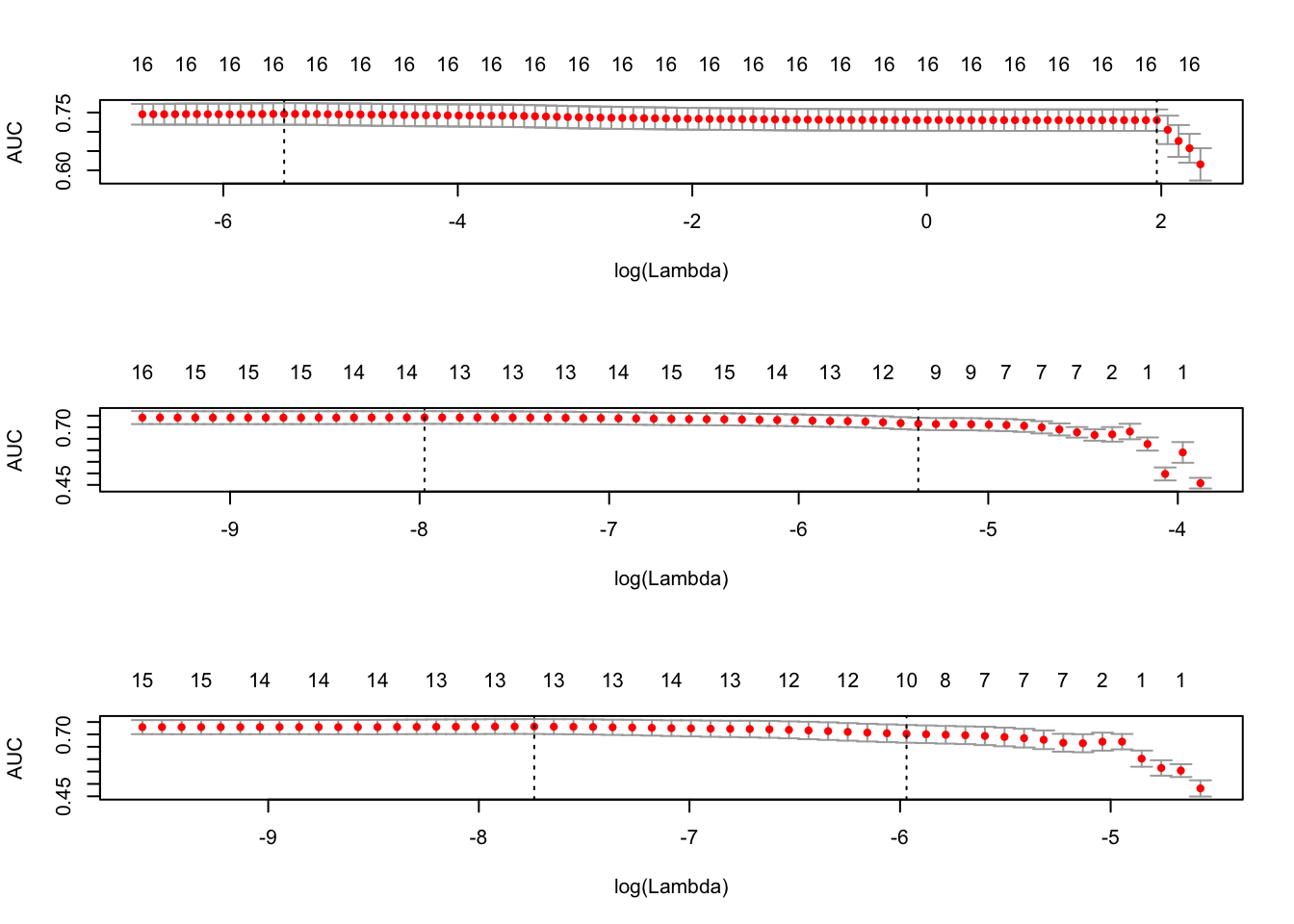## Individual Exercise Solution

Use fl2003.RData, which is a cleaned up version of the data from Fearon and Laitin (2003). Fit a model where onset is explained by all variables. Use cv.glmnet() to fit elastic net models for a variety of $$\alpha$$ values, using a loss function that is appropriate for the binomial nature of the data. Present plots of the model’s predictive accuracy for different $$\alpha$$ values. Fit a model with glmnet() using the $$\alpha$$ value you found that minimizes predictive error. Report coefficient estimates for all variables, and plot the changes in coefficient values vs. the L1 norm, log-lambda value, and deviance explained.

Randomly sample five cases where onset = 0 and five where onset = 1. Fit an elastic net with the optimal $$\alpha$$ value you found for the whole dataset. Are the most important coefficients the same?

library(dplyr)
library(glmnet)
library(caret)
library(parallel)
load('fl2003.RData')

# create predictors and response
fl_x <- as.matrix(fl[, -1])
fl_y <- as.factor(fl$onset) # sequences of alpha values to evaluate alphas <- seq(0, 1, by = .1) # cross validation elastic nets for different penalty parameters fits <- mclapply(alphas, function(x) cv.glmnet(fl_x, fl_y, type.measure = 'auc', alpha = x, family = 'binomial')) # plot AUC for different penalty parameters par(mfrow = c(3,1)) plot(fits[]) plot(fits[]) plot(fits[])# penalty parameter w/ highest AUC alpha_best <- which.max(sapply(fits, function(x) max(x$cvm)))

# fit elastic net w/ best penalty parameters
best_fit <- glmnet(fl_x, fl_y, family = 'binomial', alpha = alphas[alpha_best])

# plot coefficients
par(mfrow = c(3,1))
plot(best_fit, xvar = 'norm', label = T)
plot(best_fit, xvar = 'lambda', label = T)
plot(best_fit, xvar = 'dev', label = T)